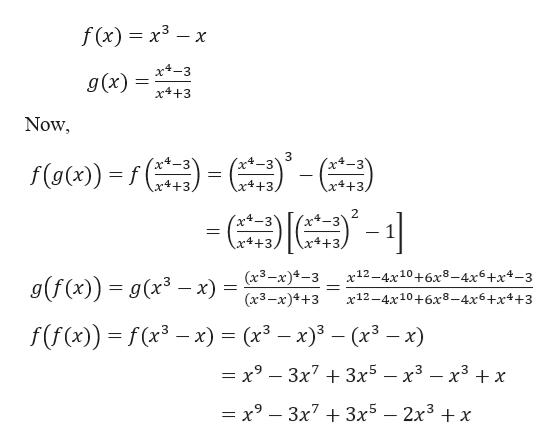# For the pair of functionsf(x)andg(x),findf(g(x)),g(f(x))andf(f(x)).f(x) = x3 − x;  g(x) = x4 − 3x4 + 3

Question
20 views

For the pair of functions

f(x)

and

g(x),

find

f(g(x)),
g(f(x))

and

f(f(x)).
f(x) = x3 − x;  g(x) =
 x4 − 3 x4 + 3
check_circle

Step 1

Given,

...help_outlineImage Transcriptionclosef (x) x3x x-3 g(x) x4+3 Now )'- (x+-3 (xt-3Y x4+3. f(o) x4 +3 x4+3 2 x-3 x4 +3, x4+3, g(f (x)) = g(x3 - x) = (3-x)4-3 (3-x)4+3 x12-4x10+6x8-4x6+x4-3 x12-4x10+6x8-4x6+x4+3 f(f(x)) f(x-x) = (x3 - x)3- (x3 — х9 — Зх7 + Зx5 — х3 — х3 +x — х9 — Зх7 + Зx5 — 2х3 + х fullscreen

### Want to see the full answer?

See Solution

#### Want to see this answer and more?

Solutions are written by subject experts who are available 24/7. Questions are typically answered within 1 hour.*

See Solution
*Response times may vary by subject and question.
Tagged in

### Functions